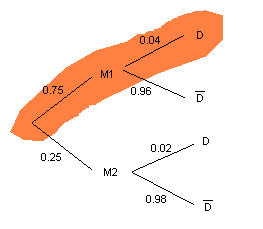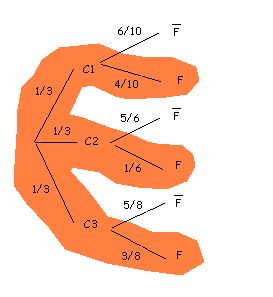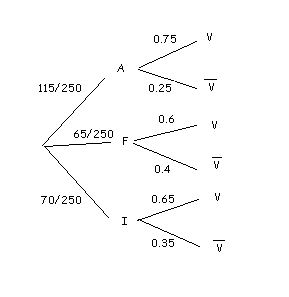# Bayes's theorem

Let's start considering the following example:

A screw factory has two machines, the $$M1$$, which is old, and does $$75\%$$ of all the screws, and the $$M2$$, newer but small, that does $$25\%$$ of the screws. The $$M1$$ does $$4\%$$ of defective screws, while the $$M2$$ just does $$2\%$$ of defective screws. If we choose a screw at random: what is the probability that ir turns out to be defectively?

As we have seen, we could solve the problem using the theorem of the total probability. Remember that it was the same as to use a tree to solve it.

Let's look now at the problem from another point of view. If we know that a screw is defective: what is the probability that it has been made by machine $$M1$$?

In other words, we are wondering for the conditional probability $$P(M1/D)$$. On the one hand, for the definition of conditional probability, we have that:

$$P(M1/D)=\dfrac{P(M1\cap D)}{P(D)}$$$On the other hand, if we represent our problem in a tree, we see that we can compute $$P(M1\cap D)$$, since it is the probability of the colored branch: to be made by $$M1$$ and to be defective.Remember that the theorem of total probability is: $$P(D) = P(M1)\cdot P(D/M1) + P(M2)\cdot P(D/M2)$$$ So we obtain: $$P(M1/D)=\dfrac{P(M1)\cdot P(D/M1)}{P(M1)\cdot P(D/M1) + P(M2)\cdot P(D/M2)}$$$In our example, $$P(M1/D)=\dfrac{0,75\cdot 0,04}{0,75\cdot 0,04 + 0,25\cdot 0,02}=0,857$$$

What we have done to solve this problem can be generalized through the Bayes' theorem.

• Bayes' Theorem:

Let $$A_1,A_2,\ldots, A_n$$ be a partition of the sample space and $$B$$ uan event associated with the same experiment. Then, we have that: $$P(A_i/B)=\dfrac{P(A_i)\cdot P(B/A_i)}{P(A_1)\cdot P(B/A_1)+\ldots+P(A_n)\cdot P(B/A_n)}$$$Let's see how to apply it. We have three boxes with light bulbs. The first one contains $$10$$ bulbs, with $$4$$ of them broken; in the second one there are $$6$$ light bulbs, and only one broken, and in the third one there are three broken light bulbs out of eight. Now we want to ask ours: If we take a broken bulb what is the probability that comes from box $$1$$? Let's remember that $$C1$$, $$C2$$, $$C3$$ represent boxes $$1$$, $$2$$ and $$3$$. Also $$F =$$ "broken bulb", for what $$\overline{F}=$$ "not broken bulb". Now, we are only interested in the top branch of our tree.We are interested in $$P(C1/F)$$. From Bayes' theorem, $$P(C1/F)=\dfrac{P(C1)\cdot P(F/C1)}{P(C1)\cdot P(F/C1)+P(C2)\cdot P(F/C2)+P(C3)\cdot P(F/C3)}$$$

In our case, $$P(C1/F)=\dfrac{\dfrac{1}{3}\cdot \dfrac{4}{10}}{\dfrac{1}{3}\cdot \dfrac{4}{10} + \dfrac{1}{3}\cdot \dfrac{1}{6}+\dfrac{1}{3}\cdot \dfrac{3}{8}}=\dfrac{\dfrac{4}{30}}{\dfrac{113}{360}}=\dfrac{48}{113}=0,425$$$that is to say, $$42,5\%$$. Suppose we have $$250$$ doctors from Europe meeting in a conference. Among these $$115$$ are Germans; $$65$$, French, and $$70$$ Englishmen. We also know that, $$75\%$$ of the Germans, $$60\%$$ of the French and $$65\%$$ of the Englishmen are in favour of using a new vaccine for the flu. In order to decide whether the vaccine is finally used they agree on the following: among all the doctors they select at random three doctors, who answer if they are in favour or not (with replacement). Remember that with replacement means that a doctor can be selected all three times (or two times). The vaccine is approved out of these three picks, at least two agree on using the vaccine. We are now interested in: If we pick a doctor randomly and he is in favor of using the vaccine: what is the probability that he is French? Let's consider the following events: $$A =$$ "German doctor", $$F =$$ "French doctor", $$I =$$ "English doctor", and $$V =$$ "to be in favor of the vaccine" (and therefore, $$\overline{V}=$$ "o be against the vaccine"). We represent our problem in a tree.We are looking for $$P(F/V)$$. From Bayes' theorem, $$P(F/V)=\dfrac{P(F)\cdot P(V/F)}{P(A)\cdot P(V/A)+P(F)\cdot P(V/F)+P(I)\cdot P(V/I)}$$$

In our case, $$P(F/V)=\dfrac{\dfrac{65}{250}\cdot 0,6}{\dfrac{115}{250}\cdot 0,75 + \dfrac{65}{250}\cdot 0,6+\dfrac{70}{250}\cdot 0,65} =0,228$$\$